# 【数电实验】随机数生成电路

## 一、设计任务要求

1. 1. 设计并实现一个随机数生成电路，每 2 秒 随机生成一个 0~999 之间的数 字，并在数码管上显示生成的随机数。
2. 2. 为系统设置一个复位键，复位后数码管显示“000”，2 秒后再开始每 2 秒 生成并显示随机数，要求使用按键复位。
3. 3. 实验板上输入时钟选择 1kHz 或更高的频率。

## 2.2模块划分## 2.3总体框图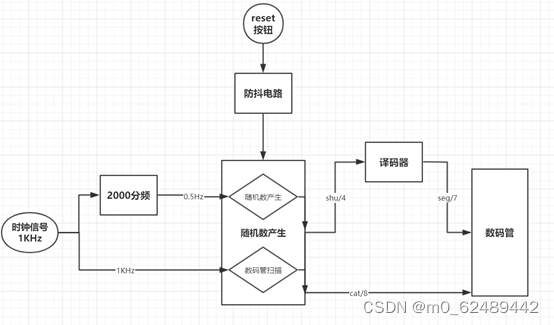## 3.1 2000分频模块

``````library ieee;                                    --库和程序包声明
use ieee.std_logic_1164.all;
use ieee.std_logic_unsigned.all;

entity div2000 is                               --2000分频器实体描述
port(
clk: in std_logic;                        --时钟输入端口
clear: in std_logic;                     --复位键输入端口
clk_out:out std_logic);                  --时钟分频后输出端口
end div2000;

architecture a of div2000 is                    --结构体描述
signal tmp: integer range 0 to 999; --整型数，用来记录分频
signal clktmp: std_logic;          --信号定义，只能是储存时钟信号
begin
process(clear,clk)
begin
if clear='1' then  --如果按下复位键，分频计数归零，重新开始计数
tmp<=0;
elsif clk'event and clk='1' then
if tmp=999 then           --从0计数到999，则信号反转一下
tmp<=0;clktmp<=not clktmp;
else
tmp<=tmp+1;
end if;
end if;
end process;
clk_out<=clktmp;
end a;
``````

## 3.2 防抖模块

``````library ieee;                                     --库和程序包声明
use ieee.std_logic_1164.all;

entity antisk is                                --防抖电路实体描述
port(clk:in std_logic;                      --时钟信号
input:in std_logic;                     --按钮输入端口
output:out std_logic                   --输出端口
);
end antisk;

architecture a of antisk is
signal a:std_logic;
signal count:integer range 0 to 9;
begin
process(clk)
begin
if input='0' then
count<=0;
elsif (clk'event and clk='1') then              --当按钮输入时
if count=9 then                     --当按钮按动持续一定时间
count<=count;
else count<=count+1;
end if;
end if;

if count=8 then a<='1';                --则输出为正
else a<='0';
end if;
end process;
output<=a;
end;
``````

## 3.3 随机数生成模块

``````library ieee;                                   --库和程序包
use ieee.std_logic_1164.all;
use ieee.std_logic_unsigned.all;
use ieee.std_logic_arith.all;

entity random is
port (clk1000:in std_logic;                       --时钟输入1KHz
clk2:in std_logic;                           --时钟输入0.5Hz
clear:in std_logic;                           --复位
f1_out:std_logic_vector(3 downto 0); --第一个随机数（为了波形能看到）
f2_out:std_logic_vector(3 downto 0); --第二个随机数（为了波形能看到）
f3_out:std_logic_vector(3 downto 0); --第三个随机数（为了波形能看到）
shu:out std_logic_vector(3 downto 0);--送给译码电路译码
cat:out std_logic_vector(7 downto 0));--数码管亮还是不亮控制
end entity;

architecture a of random is
signal tmp:integer range 0 to 2;         --用来循环让三个数码管依次亮起
signal f:std_logic_vector(5 downto 0);       --计数增加
signal f0:std_logic_vector(5 downto 0); --从f中取数，供以产生三个随机数
signal f1:std_logic_vector(3 downto 0);      --第一个随机数
signal f2:std_logic_vector(3 downto 0);      --第一个随机数
signal f3:std_logic_vector(3 downto 0);      --第一个随机数

begin
process(clk1000,clk2,clear)     --开始进程，以时钟clk1000,
begin                          --和时钟clk2，复位清除clear为敏感信号
if (clk2'event and clk2='1') then f<=f+3; end if;   --f每2秒变一回

if clear='1' then f0<="000000";            --当复位信号来了，f0赋值为0
elsif (clk2'event and clk2='1') then    --如果复位信号没有来，每两秒
f0<=f;                               --将f的值赋给f0
end if;

f1(0)<=f0(0) xor f0(1);   --第一位数的第0位是f0的第0位与第1位异或
f1(1)<=f0(1) xor f0(2);   --第一位数的第1位是f0的第1位与第2位异或
f1(2)<=f0(2) xor f0(3);   --第一位数的第2位是f0的第2位与第3位异或
f1(3)<=f0(3) xor f0(0);   --第一位数的第3位是f0的第3位与第0位异或

f2(0)<=f0(1) xor f0(2);   --第二位数的第0位是f0的第1位与第2位异或
f2(1)<=f0(2) xor f0(3);   --第二位数的第0位是f0的第2位与第3位异或
f2(2)<=f0(3) xor f0(4);   --第二位数的第0位是f0的第3位与第4位异或
f2(3)<=f0(4) xor f0(1);   --第二位数的第0位是f0的第4位与第1位异或

f3(0)<=f0(2) xor f1(3);   --第三位数的第0位是f0的第2位与第3位异或
f3(1)<=f0(3) xor f0(4);   --第三位数的第1位是f0的第3位与第4位异或
f3(2)<=f0(4) xor f0(5);   --第三位数的第2位是f0的第4位与第5位异或
f3(0)<=f0(5) xor f0(2);   --第三位数的第3位是f0的第5位与第2位异或

if (clk1000'event and clk1000='1') then  --用来判断刷新三个数码管
if tmp=2 then tmp<=0 ;else tmp<=tmp+1;--刷新的频率大于50Hz
end if;end if;                           --人眼看到就不会抖动

case tmp is
when 0=> shu<=f1;cat<="11110111";    --tmp=0，第3个数码管亮
when 1=> shu<=f2;cat<="11111011";    --tmp=1，第2个数码管亮
when 2=> shu<=f3;cat<="11111101";    --tmp=2，第1个数码管亮
end case;

f1_out<=f1;f2_out<=f2;f3_out<=f3;     --看最后总体的输出波形使用
end process;

end;
``````

## 3.4 数码管译码电路

``````LIBRARY IEEE;
USE IEEE.STD_LOGIC_1164.ALL;

ENTITY seg IS
PORT(
a:IN STD_LOGIC_VECTOR(3 DOWNTO 0);    --输入端口
b:OUT STD_LOGIC_VECTOR(6 DOWNTO 0));  --输出端口
END seg;

ARCHITECTURE seg7_1_arch OF seg IS
BEGIN

PROCESS(a)
BEGIN
CASE a IS
WHEN "0000" => b <= "1111110";  --输入是0，则显示0
WHEN "0001" => b <= "0110000";  --输入是1，则显示1
WHEN "0010" => b <= "1101101";  --输入是2，则显示2
WHEN "0011" => b <= "1111001";  --输入是3，则显示3
WHEN "0100" => b <= "0110011";  --输入是4，则显示4
WHEN "0101" => b <= "1011011";  --输入是5，则显示5
WHEN "0110" => b <= "1011111";  --输入是6，则显示6
WHEN "0111" => b <= "1110000";  --输入是7，则显示7
WHEN "1000" => b <= "1111111";  --输入是8，则显示8
WHEN "1001" => b <= "1111011";  --输入是9，则显示9

WHEN "1010" => b <= "1110000"; --输入是10，则显示7
WHEN "1011" => b <= "0110000"; --输入是11，则显示1
WHEN "1100" => b <= "0110011"; --输入是12，则显示4
WHEN "1101" => b <= "1111111"; --输入是13，则显示8
WHEN "1110" => b <= "1011111"; --输入是14，则显示6
WHEN "1111" => b <= "1111110"; --输入是15，则显示0
END CASE;
END PROCESS;
END;
``````

## 3.5 总体电路连接模块

``````library ieee;
use ieee.std_logic_1164.all;
use ieee.std_logic_unsigned.all;
use ieee.std_logic_arith.all;
entity whole is
port (clock,key:in std_logic;             --时钟和复位信号
seg0:out std_logic_vector(6 downto 0); --数码管
shu:out std_logic_vector(3 downto 0); --数码管此时译码的数
f1:out std_logic_vector(3 downto 0); --第一个随机数（为了波形看见）
f2:out std_logic_vector(3 downto 0); --第一个随机数（为了波形看见）
f3:out std_logic_vector(3 downto 0); --第一个随机数（为了波形看见）
cat0:out std_logic_vector(7 downto 0));--数码管显示控制
end entity whole;

architecture a of whole is
component div2000                        --2000分频器组件声明
port(
clk: in std_logic;
clear: in std_logic;
clk_out:out std_logic);
end component;

component random                         --随机数组件声明
port (
clk1000:in std_logic;
clk2:in std_logic;
clear:in std_logic;
f1_out:out std_logic_vector(3 downto 0);
f2_out:out std_logic_vector(3 downto 0);
f3_out:out std_logic_vector(3 downto 0);
shu:out std_logic_vector(3 downto 0);
cat:out std_logic_vector(7 downto 0));
end component;

component antisk                              --防抖电路组件声明
port(clk:in std_logic;
input:in std_logic;
output:out std_logic);
end component;

component seg                            --译码电路组件声明
port (
a:IN STD_LOGIC_VECTOR(3 DOWNTO 0);
b:OUT STD_LOGIC_VECTOR(6 DOWNTO 0));
END component;

signal clea,cp1000,cp2:std_logic;
signal yi:std_logic_vector(3 downto 0);

begin                                             --电路连接
shu<=yi;
u1:div2000 port map (clk=>clock,clear=>clea,clk_out=>cp2);
u2:antisk port map(clk=>clock,input=>key,output=>clea);
u3:random
port map
(clk1000=>clock,clk2=>cp2,clear=>clea,cat=>cat0,shu=>yi,f1_out=>f1,f2_out=>f2,f3_out=>f3);
u4:seg port map (a=>yi,b=>seg0);
end;
``````

## 4.1 2000分频器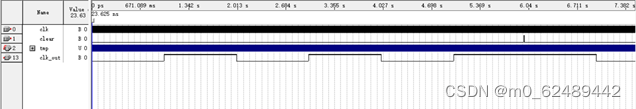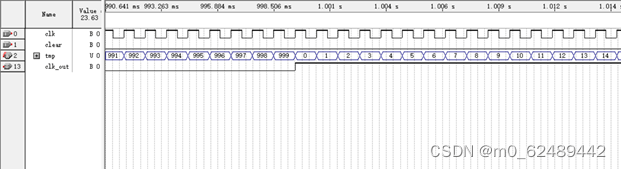图2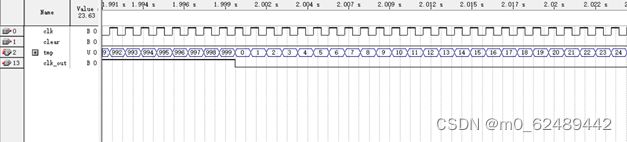图3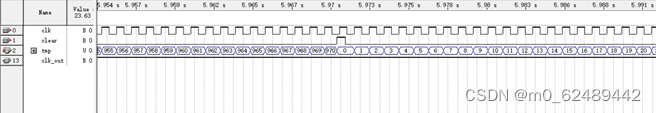图4

## 4.2 防抖电路模块图1图2

## 4.3 随机数生成模块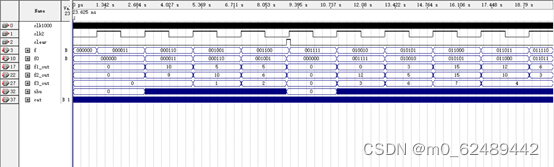图1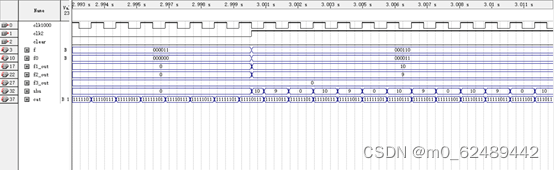图2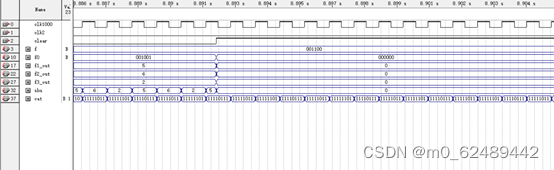图3

## 4.4 译码电路模块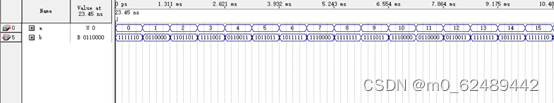## 4.5 电路综合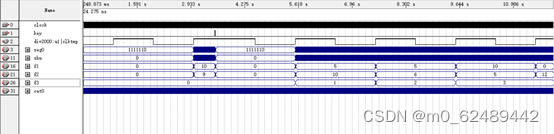图1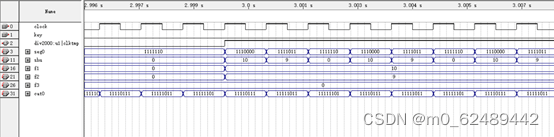图2

# 五、故障及问题分析

1. 最后电路综合仿真的时候，想要出现的引脚没有。解决方法，最后加上一个输出引脚，这样最后的仿真波形的选项最后就能出现所要选择的。
2. 随机数生成电路，最开始设计的时候一按复位键，然后出现的数字都是固定的。要解决这样的问题，可以把计数信号，和给最后的进行运算变换的信号分开，这样即使运算变换的信号变为0，计数信号不是0，这样最后就能达到复位之后，下一个数字不是0的效果。
3. 译码的时候要考虑到二进制和十进制之间的区别，把所有的情况都考虑一遍，就是把10~15的情况思考清楚。